# KCET 2020 Chemistry Paper with Solutions

Students can download the KCET 2020 Question Paper with solutions for the Chemistry section from this page. The questions have been solved by our expert teachers and the solutions have been prepared in a detailed manner. This has been done to address the need of students to find the right answer to each of the questions asked in KCET 2020 Paper. The questions and solutions can be either viewed on our page directly or the document which is available in a PDF format can be downloaded for offline use. Practising KCET 2020 Chemistry Question Paper will help the candidates to study productively and at the same time analyze their performance in a more practical manner. Ultimately, aspirants will be able to face the exam with higher confidence and score more marks in the upcoming KCET exam.

### KCET 2020 - Chemistry

Question 1. Aqueous solution of a salt (A) forms a dense white precipitate with BaCl2 solution. The precipitate dissolves in dilute HCl to produce a gas (B) which decolourises acidified KMnO4 solution. A and B respectively are:

1. a. BaSO3, H2S
2. b.BaSO4, SO2
3. c. BaSO3, SO2
4. d. BaSO4, H2S

Solution:

The corresponding reactions are

BaCl2 + Na2 SO3 → BaSO3 + 2NaCl

BaSO3 + 2HCl → BaCl2 + SO2 + H2 O

Salt A is Na2SO3 and the dense white precipitate is BaSO3 (Barium sulfite).

Gas B is SO2. It decolourises acidified KMnO4 solution because of its reducing nature.

The corresponding reaction is:

2KMnO4 + 5SO2 + 2H2 O → K2 SO4 + 2MnSO4 + 2H2 SO4

Question 2. Bond angle in PH4+ is more than that of PH3. This is because:

1. a. PH3 has a planar trigonal structure
2. b. Hybridisation of P changes when PH3 is converted to PH4+
3. c. Lone pair-bond pair repulsion exists in PH3
4. d. PH4+ has a square planar structure

Solution:

The hydrides of group 15, 16 below the 3rd period, follows Drago’s rule. The rule states that due to a large energy difference between the atomic orbitals, these compounds do not exhibit hybridization. Thus, PH3 will not exhibit hybridization and here the bond formation takes place due to the overlap of pure p-orbitals and s-orbitals. PH3 has a lone pair on the central P atom, which is absent in PH4+. Thus in PH3, there will be bond pair – lone pair repulsion and this is the reason why the bond angle in PH3 is less than that of PH4+.

Question 3. Incorrectly matched pair is:

1. a. XeF6 – distorted octahedral
2. b. XeOF4 – square pyramidal
3. c. XeO3 – pyramidal
4. d. XeF4 – tetrahedral

Solution:

Solution:: (d)

In XeF6 there are 6 bond pairs and one lone pair. Thus the shape of XeF6 is distorted octahedral.

In XeOF4, Xe is sp3d hybridized and the shape is square pyramidal.

In XeO3, Xe is sp3 hybridized and the shape is trigonal pyramidal.

In XeF4, Xe is sp3 d2 hybridized. It has 4 bond pairs and two lone pairs. Thus, the shape issquare planar.

Question 4. Phosphorous pentachloride

1. a. Has all the five equivalent bonds
2. b. Exist as an ionic solid in which the cation has an octahedral structure and the anion has a tetrahedral structure
3. c. On hydrolysis gives an oxo acid of phosphorous which is tribasic
4. d. On hydrolysis gives an oxo acid of phosphorous which is a good reducing agent

Solution:

(a) Phosphorous pentachloride (PCl5) has a trigonal bipyramidal structure. Here, the five bonds are not of equal lengths as the axial bonds are slightly longer than the equatorial bonds.

(b) PCl5 solid exists as [PCl4]+ and [PCl6]- and they have tetrahedral and octahedral structures respectively.

(c) On hydrolysis, PCl5 gives H3 PO4which is tribasic.

PCl5 + 4H2 O →H3 PO4 + 5HCl

In H3PO4, P is in its +5 oxidation state. So it is in its highest oxidation state and hence is not a good reducing agent.

Question 5. Identify the set of paramagnetic ions among the following:

1. a. Ti3+, Cu2+, Mn3+
2. b. Sc3+, Ti3+, V3+
3. c. V2+, Co2+, Zn2+
4. d. Ni2+,Cu2+,Zn2+

Solution:

The ions which contain unpaired electrons exhibit paramagnetic behaviour.

The electronic configuration of the ions are given below:

Ti3+=1s2 2s2 2p6 3s2 3p5

Cu2+=1s2 2s2 2p6 3s2 3p5 3d9

Mn3+=1s2 2s2 2p6 3s2 3p5 3d4

Thus, all of them have unpaired electrons. Hence, they will show paramagnetic behaviour.

In option (B) V3+ does not possess any unpaired electrons.

In option (C) Zn2+ does not possess any unpaired electrons.

Similarly, in option (D) Zn2+ does not possess any unpaired electrons.

Question 6. How many moles of K2(Cr)2O7 is required to liberate 6 moles of I2 from an aqueous solution of I-?

1. a. 0.25
2. b. 0.5
3. c. 2
4. d. 1

Solution:

The balanced redox reaction will be: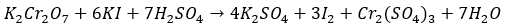3 moles of I2 requires 1 mole of K2Cr2O7

Hence, 6 moles of I2 will require 2 moles of K2Cr2O7

Question 7. Cu2Cl2 and CuCl2 in an aqueous medium

1. a. Both are unstable
2. b. Cu2Cl2is more stable than CuCl2
3. c. CuCl2is more stable than Cu2 Cl2
4. d. Stability of Cu2Cl2 is equal to the stability of CuCl2
5. d.pends on the size of the cation. Smaller the size of the cation more will be the hydration

enthalpy. Hence, more will be stability.

Solution:

CuCl2 is more stable than Cu2 Cl2. The size of Cu2+ is smaller than Cu+. The hydration enthalpy

d.pends on the size of the cation. Smaller the size of the cation more will be the hydration

enthalpy. Hence, more will be stability.

Question 8. The co-ordination number of Fe and Co in the complex ions [Fe(C2O4 )3]3- and [Co(SCN)4]2- are respectively:

1. a. 4 and 6
2. b. 6 and 4
3. c. 3 and 4
4. d. 6 and 8

Solution:

C2 O42- is the oxalate ligand. It binds through two oxygen atoms and thus, is a bidentate ligand.

Hence the co-ordination number of Fe in [Fe(C2 O4 )3]3- is 6.

SCN- is the thiocyanate ligand. It is a monodentate ligand. Hence the co-ordination number of

Co in the complex ion [Co(SCN)4]2- is 4.

Question 9. Number of stereoisomers exhibited by [Co(en)2Cl2]+ is

1. a. 5
2. b. 3
3. c. 4
4. d. 2

Solution: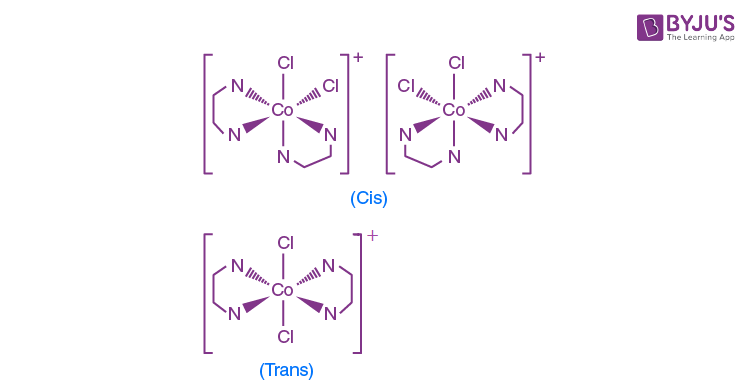Question 10. The IUPAC name of [Pt(NH3 )4 ][PtCl4] is:

1. a. Tetra ammine palatinate (0) tetra chloride platinum (IV)
2. b. Tetra ammine platinum (II) tetra chloride palatinate (II)
3. c. Tetra ammine platinum (0) tetra chloride platinum (IV)
4. d. Tetra ammine palatinate (II) tetra chloride platinum (II)

Solution:

The coordination complex must be neutral. In all the options, the oxidation states of Pt are given such that we get an overall neutral complex. Again, while naming a complex, the cationic part should be named first followed by the anionic part. While naming the anionic part, the name of the metal should end with the suffix –ate. From this, we can conclude that the IUPAC name of [Pt(NH3 )4 ][PtCl4] can be either (A) or (D). But the most common oxidation state of Pt is +2 and +4 and in both the parts of the complex, Pt has the same coordination number, and so, the correct option will be (D).

Question 11. Prolonged exposure of chloroform in humans may cause damage to the liver. It is due to the formation of the following compound:

1. a. CH2 Cl2
2. b. Cl2
3. c. CCl4
4. d. COCl2

Solution:

When chloroform is oxidised, it forms phosgene which is poisonous.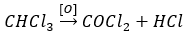Question 12. Which of the following halides show the highest reactivity towards SN1 reactions?

1. a. CH3-CH2-CH2-CH2 I
2. b. C6 H5 Cl
3. c. C6 H5 CH2 Cl
4. d. CH3 CH2 Cl

Solution:

The rate of an SN 1 reaction is determined by the stability of the intermediate formed. In this case, the most stable intermediate formed would be for C6 H5 CH2 Cl and the intermediate formed would be C6 H5 CH2+. This intermediate is stabilised via resonance.

Question 13. In the reaction: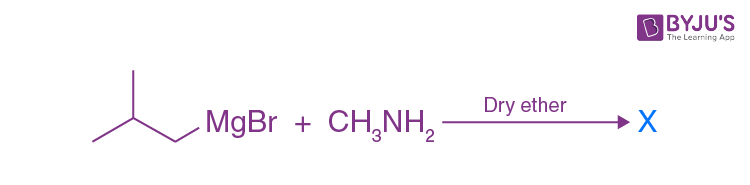The number of possible isomers for compound X is:

1. a. 3
2. b. 2
3. c. 4
4. d. 5

Solution: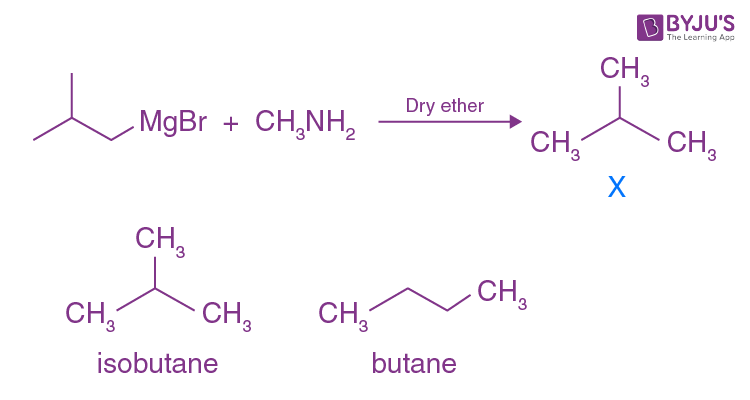Question 14. Which of the following on heating gives ether as the major product?

P C6 H5 CH2 Br + CH3ONa

Q C6 H5ONa + CH3 Br

R (CH3 )3 C-Cl + CH3ONa

S C6 H5 CH=CHCl+CH3ONa

1. a. Both Q and S
2. b. Both P and Q
3. c. Both R and S
4. d. Both P and R

Solution:

Primary alkyl halides/ benzyl halides react with alkoxide/phenoxide to give ethers via SN2 mechanism. This reaction is known as Williamson’s ether synthesis. Aryl halides are the least reactive as the C-X bond has a partial double bond characteristic and hence, does not cleave easily. 3o halides do not give ethers as they undergo elimination in the presence of a strong base like alkoxides.

Question 15. The steps involved in the conversion of propan-2-ol to propan-1-ol are in the order:

1. a. Heating with PCl5, heating with alc.KOH, hydroboration-oxidation
2. b. Dehydration, addition of HBr in the presence of a peroxide, heating with alc.KOH
3. c. Dehydration, addition of HBr, heating with alc.KOH
4. d. Heating with PCl5, heating with alc.KOH, acid catalysed addition of water

Solution: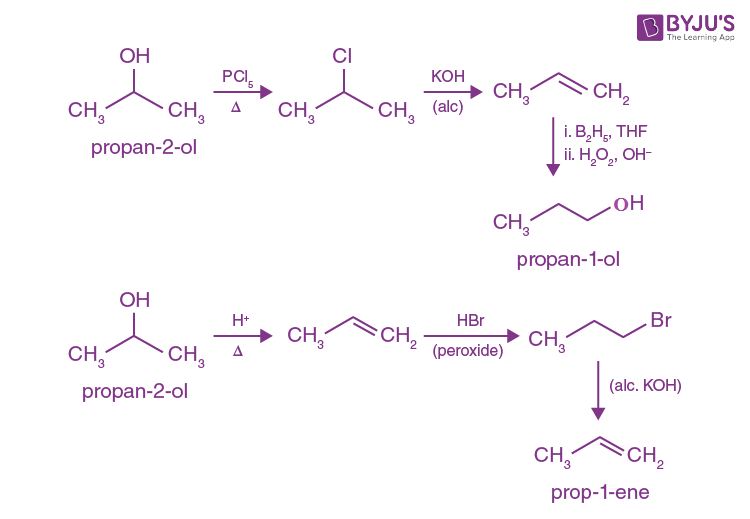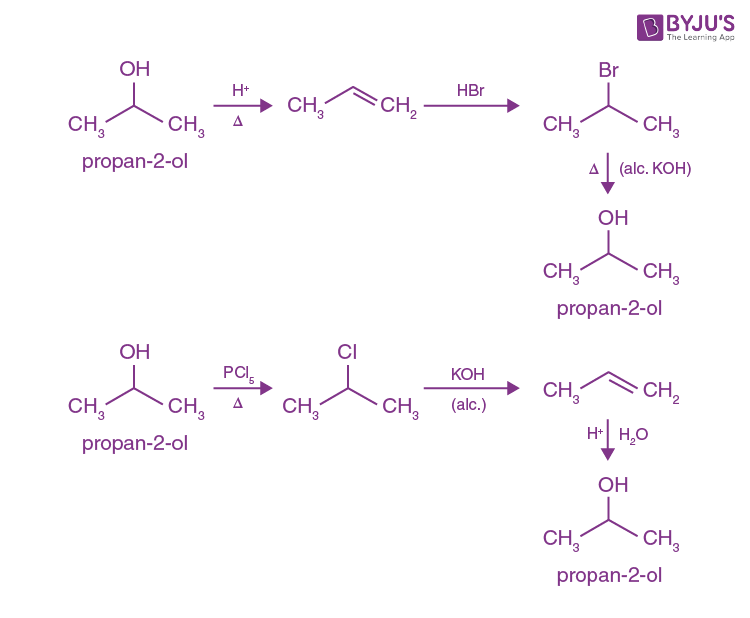Question 16. Which of the following is the strongest base?

1. a. OH-
2. b. CH3 O-
3. c. CH3 COO-
4. d. Cl-

Solution:

To check the basicity of an ion, the conjugate acid must be weak. The conjugate acids are given below: H2O, CH3 OH, CH3 COOH and HCl.

We know that methanol is a stronger acid than water. Weaker the acid, stronger is the conjugate base, by that logic, OH- is the strongest base among the given options.

Question 17. Product ‘P’ is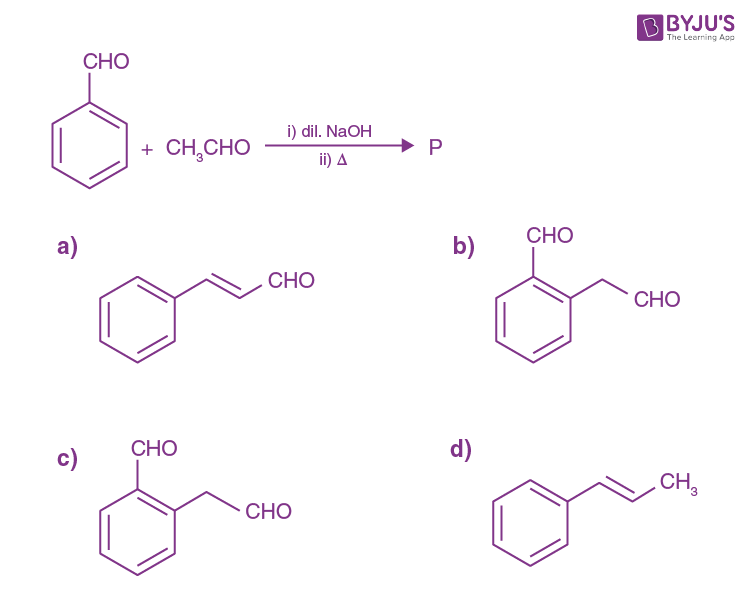Solution: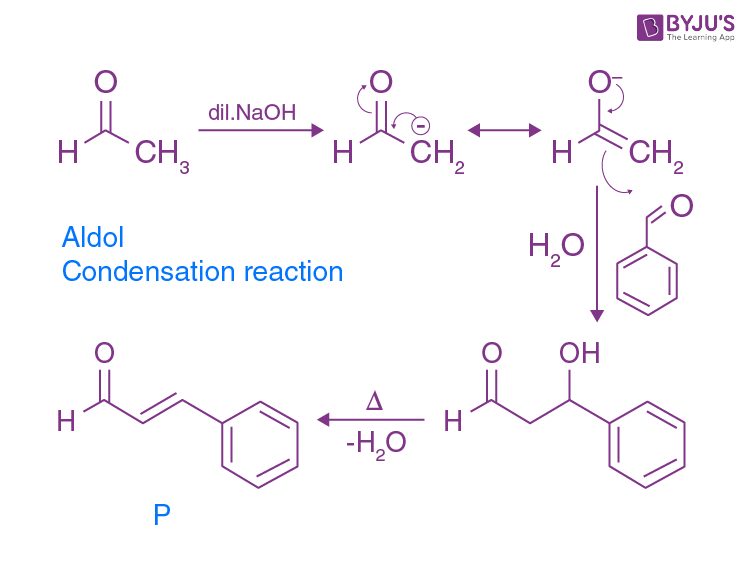Question 18. Which of the following has the lowest boiling point?

1. a. CH3-O-CH3
2. b. HCOOH
3. c. CH3 CH2 OH
4. d. CH3 CH2 NH2

Solution:

Ethers cannot form strong hydrogen bonds and hence they have the lowest boiling point among the given options.

Question 19. The carbonyl compound that does not undergo aldol condensation is:

1. a. Trichloroacetaldehyde
2. b. Acetaldehyde
3. c. Acetone
4. d. Dichloroacetaldehyde

Solution:

At least one α-hydrogen should be present for an aldehyde or a ketone to undergo aldol reaction. The only compound that does not have even one α-hydrogen is trichloroacetaldehyde, and hence, it does not undergo aldol reaction.

Question 20. In the following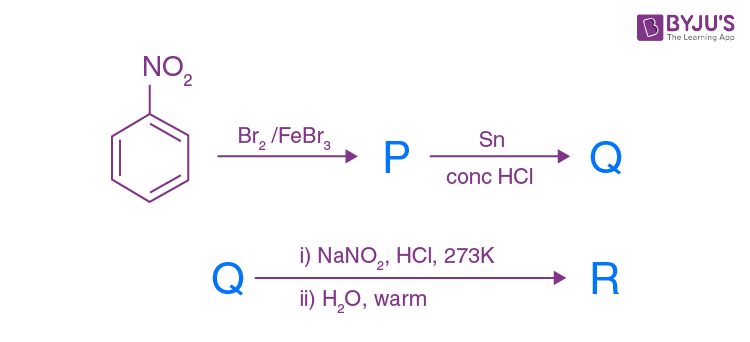The final product R is: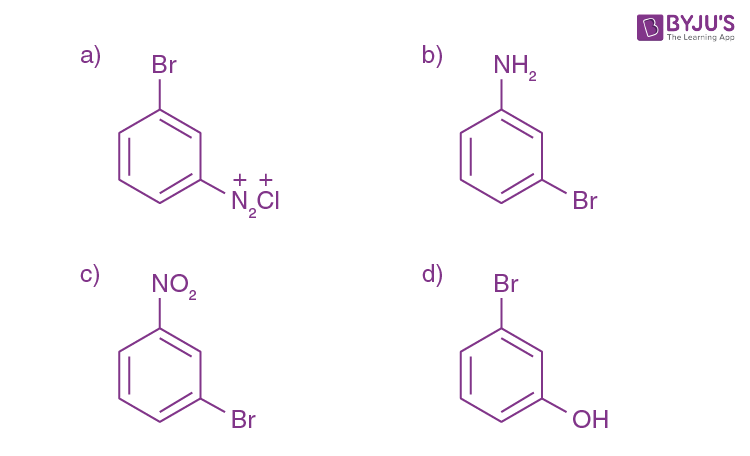Solution: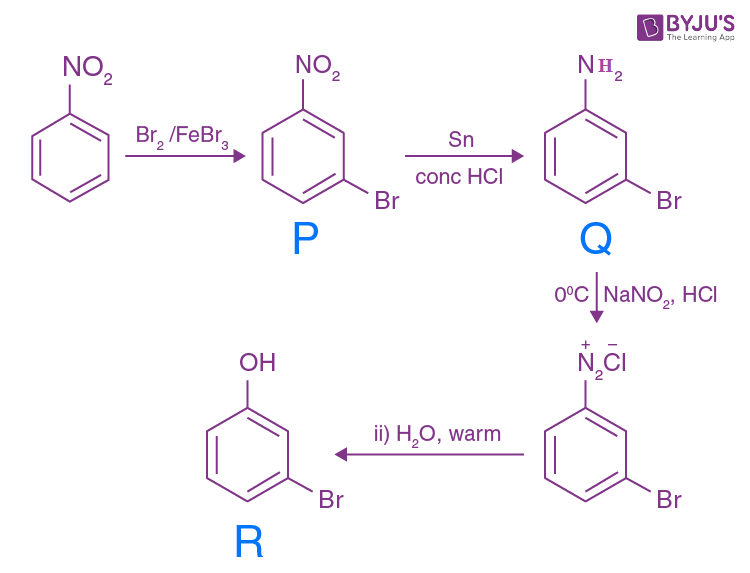Question 21. Hinsberg’s reagent is:

1. a. C6H5SO2 NH2
2. b. CH3COCl/pyridine
3. c. (CH3CO)2 O/pyridine
4. d. C6H5SO2Cl

Solution:

Hinsberg’s reagent is benzene sulphonyl chloride (C6 H5 SO2 Cl).

It is used to test for and distinguish between primary, secondary and tertiary amines.

Question 22. Which of the following vitamins is not stored in the adipose tissue?

1. a. D
2. b. E
3. c. A
4. d. B6

Solution:

Adipose tissue stores those vitamins which are fat-soluble. Among the given vitamins, only A, D and E are fat-soluble. Vitamin B6 is water-soluble and hence, is not stored in adipose tissue.

Question 23. Hypothyroidism is caused by the deficiency of:

1. a. Thyroxine
2. b. Glucocorticoid
3. c. Vitamin B-12

Solution:

Hypothyroidism is caused by the deficiency of the hormone thyroxine which is produced by the thyroid gland.

1. a. Lactose
2. b. Starch
3. c. Maltose
4. d. Sucrose

Solution: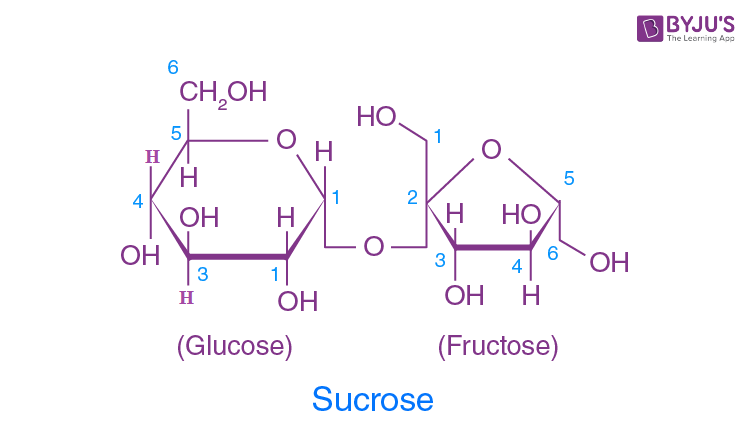Sucrose has C1-C2 glycosidic bond and notC1-C4 glycosidic bond.

Question 25. Which of the following polymers has the strongest intermolecular forces of attraction?

1. a. Polythene
2. b. Polystyrene
3. c. Neoprene
4. d. Terylene

Solution:

The monomer of polythene is ethane (C2 H4 )

The monomer of polystyrene is styrene (C6 H5 CHCH2 )

The monomer of neoprene is chloroprene (CH2=C(Cl)-CH=CH2 )

The monomer of Terylene is 1,4 –benzene dicarboxylic acid and 1,2-ethanediol. Thus, it possesses intermolecular hydrogen bonding and has the strongest intermolecular forces among the given options.

Question 26. Which of the following monomers can undergo condensation polymerisation?

1. a. Isoprene
2. b. Propene
3. c. Styrene
4. d. Glycine

Solution:

Glycine can undergo condensation polymerisation. Given below is an example: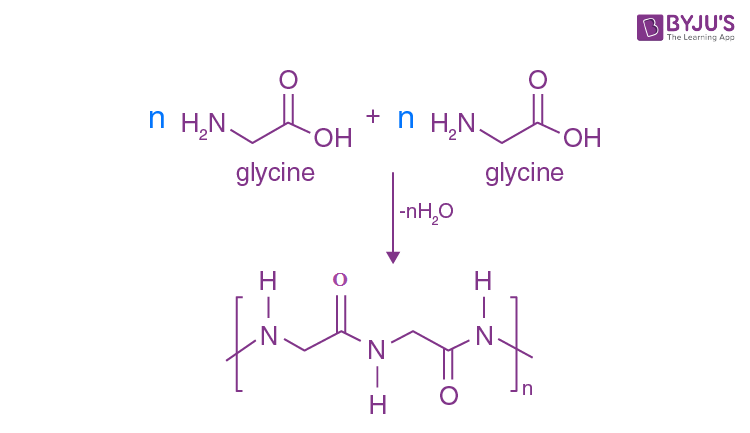Question 27. A food additive that also acts as an anti-oxidant is:

1. a. Sugar syrup
2. b. Salt
3. c. BHA
4. d. Saccharin

Solution:

BHA (Butylated hydroxyl anisole) is widely used as an anti-oxidant in foods like vegetable oils to stop them from getting oxidised and thereby, preventing them from going rancid.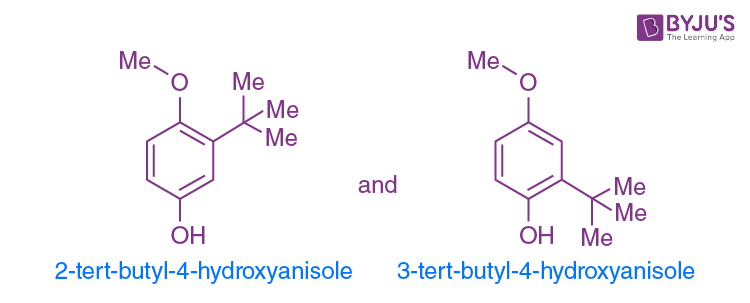Question 28. Which of the following is not related to drug-enzyme interaction?

1. a. Co-enzymes
2. b. Enzyme inhibitor
3. c. Allosteric site
4. d. Antagonist

Solution:

Antagonists impede the action of drugs by occupying the enzyme sites themselves and hence is the odd one out.

Question 29. 0.4 g of dihydrogen is made to react with 7.1 g of dichloride to form hydrogen chloride. The volume of hydrogen chloride formed at 273 K and 1 bar pressure is:

1. a. 90.8 L
2. b. 45.4 L
3. c. 9.08 L
4. d. 4.54 L

Solution:

The corresponding balanced reaction is:

H2+Cl2→2HCl

Again, 0.4 g H2 = 0.4/2 mol = 0.2 mol

7.1 g Cl2 = 7.1/71 mol = 0.1 mol

So, Cl2 is the limiting reagent here.

Hence 0.2 moles of HCl will be formed.

Again, we need to find out the volume of HCl formed in the STP condition.

1 mol of gas in STP occupies 22.7 L

Thus, 0.2 mol of HCl will occupy = 4.54 L

Question 30. With regard to the photoelectric effect, identify the correct statement among the following:

1. a. Number of electrons ejected increases with the increase in work function
2. b. Number of electrons ejected increases with the increase in the intensity of incident light
3. c. Energy of ejected electrons increases with the increase in the intensity of incident light
4. d. Numbers of electrons ejected increases with the increase in the frequency of the incident light

Solution:

Photoelectric effect is the emission of electrons from a certain metal surface when it is irradiated with photons or light. The minimum frequency required to eject an electron from a metal surface is called threshold frequency. (υo)

The equation for the photoelectric effect is:

Hυ = hυo+KE , where υ = frequency of the incident radiation

Here hυo is the work function. The number of electrons ejected does not depend on work function.

The number of electrons ejected increases with the increase in the intensity of incident light, an increase in the intensity of incident light means that the number of photons incident per unit surface area of the metal increases. (Provided the incident photons has its frequency more than the threshold frequency)

The energy of the ejected electrons increases with an increase in the frequency of incident light.

Question 31. The last element of the p-block in the 6th period is represented by the outermost electronic configuration:

1. a. 4f14 5d106s26p4
2. b. 4f14 5d106s2 6p6
3. c. 7s2 7p6
4. d. 4f14 6d107s2 7p6

Solution:

The last element of the p-block in 6th period is Radon (Rn)

The electronic configuration of Rn is [Xe]4f14 5d106s2 6p6

Question 32. The conjugate base of NH3 is:

1. a. NH2 OH
2. b. NH2-
3. c. NH4+
4. d. NH4 OH

Solution:

A conjugate base has one proton less from the acid. If we remove one proton from NH3, we get NH2-. NH3→NH2-+H+.

Question 33. A gas mixture contains 25% He and 75% CH4 by volume at a given temperature and pressure. The percentage by mass of methane in the mixture is approximately:

1. a. 92%
2. b. 8%
3. c. 75%
4. d. 25%

Solution:

Since temperature and pressure are constant, we can assume it to be in STP condition.

Now, let the total volume be 100 L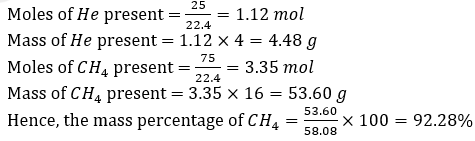Question 34. The percentage of s–character in the hybrid orbitals of nitrogen in NO2+, NO3- and NH4+ respectively are:

1. a. 50%, 33.3%, 25%
2. b. 25%, 50%, 33.3%
3. c. 33.3%, 50%, 25%
4. d. 33.3%, 25%, 50%

Solution:

In NO2+, the steric number of N is two (two sigma bonds). Thus, it is sp hybridized. Hence, the percentage s-character is = ½ ×100=50%.

In O3-, the steric number of N is three (three sigma bonds). Thus, it is sp2 hybridized. Hence the percentage s-character is = 1/3×100=33.33%.

In NH4+, the steric number of N is four (four sigma bonds). Thus, it is sp3 hybridized.

Hence, the percentage s-character is = 1/4 ×100=25%

Question 35. The formal charge on the central oxygen atom in ozone is:

1. a. +2
2. b. +1
3. c. -1
4. d. 0

Solution:(number of valence electron(s) in the neutral atom) - 1/2 (number of valence electron(s) in the

covalent bond)-(number of electrons in lone pair)

6-1/2×6 – 2=+1

Formal charge on the central oxygen i.e. oxygen (1) is +1.

Question 36. When the same quantity of heat is absorbed by a system at two different temperatures T1 and T2, such that T1>T2, change in entropies are ∆S1 and ∆S2 respectively. Then:

1. a. S2>S1
2. b. ∆S2<∆S1
3. c. ∆S1<∆S2
4. d. ∆S2 = ∆S1

Solution:

In thermodynamics, we are concerned about entropy change due to the transfer of heat.

Which we can write in the form, ∆S = q/T, here q is constant.

So, ∆S ∝ 1/T

We are given, T1>T2, so the actual relation between the entropies will be ∆S1<∆S2.

Question 37. The oxidation number of nitrogen atoms in NH4 NO3 are:

1. a. +3,-5
2. b. -3,-3
3. c. +5,-5
4. d. -3,+5

Solution:

NH4 NO3 is a salt. The cationic part is NH4+ and the anionic part is NO3-.

In NH4+, let the oxidation number be x. So,

x+4 = 1

⇒x = -3

In NO3-, let the oxidation number be y. So,

y-6 = -1

⇒y = +5

Question 38. A Lewis acid `X’ reacts with LiAlH4 in ether medium to give a highly toxic gas. This gas when heated with NH3 gives a compound commonly known as inorganic benzene. The gas is:

1. a. B3 N3 H6
2. b. BF3
3. c. B2 O3
4. d. B2 H6

Solution:

Question 39. The oxide of potassium that does NOT exist is:

1. a. K2 O2
2. b. K2 O3
3. c. K2 O
4. d. KO2

Solution:

Potassium generally forms three oxides: K2 O, K2 O2 and KO2.

K2 O3 is not formed in normal conditions and hence, is the answer.

Question 40. The metal that produces H2 with both dil. HCl and NaOH (aq) is:

1. a. Ca
2. b. Fe
3. c. Zn
4. d. Mg

Solution:

Zn produces H2 with both dil. HCl and NaOH (aq). The corresponding reactions are:

Zn+2HCl → ZnCl2+H2

Zn+2NaOH →Na2 ZnO2+H2

Question 41. Which of the following is not a pair of functional isomers?

1. a. CH3 CH2 NO2and H2 NCH2 COOH
2. b. CH3 COOH and HCOOCH3
3. c. C2 H5 OC2 H5and C3 H7 OCH3
4. d. CH3 CH2 OH and CH3 OCH3

Solution:

Functional isomers are compounds having the same molecular formula but different functional groups. Both the compounds given in option c are ethers. C2 H5 OC2 H5 and C3 H7 OCH3 are metamers, while the rest of the options are examples of functional isomers.

Question 42. Identify ‘X’ in the following reaction: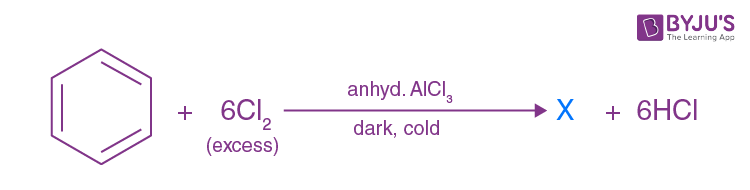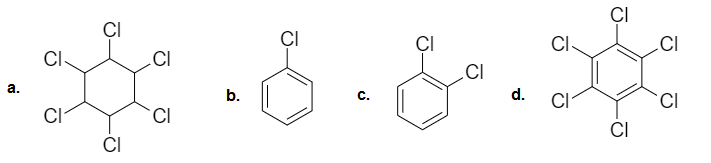Solution: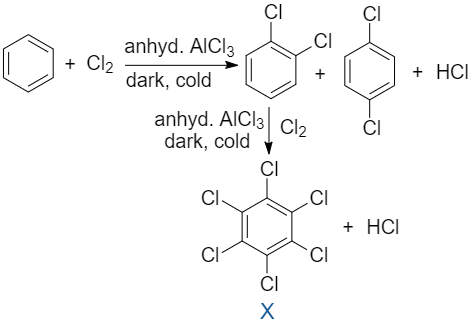Question 43. Which of the following is NOT a greenhouse gas?

1. a. O2
2. b. NO2
3. c. CFC
4. d. CO2

Solution:

Greenhouse gases are those compounds which increase the temperature of the atmosphere by absorbing the IR rays of the sun. They include compounds like water vapour, CO2, NO2, O3, CFCs etc. O2 is not a greenhouse gas.

Question 44. A metal exists as an oxide with the formula M0.96 O. Metal M can exist as M2+ and M3+ in its oxide M0.96O. The percentage of M3+in the oxide is nearly:

1. a. 5%
2. b. 9.6%
3. c. 8.3%
4. d. 4.6%

Solution:

Let, the number of M2+ ions = x

Then, the number of M3+ ions will be 0.96 – x

We know, the overall charge in the metal oxide is zero.

So, x(2)+(0.96 – x)(3)+1(-2)= 0

⇒ 2x + 2.88 – 3x = 2

⇒ -x = -0.88

⇒ x = 0.88

∴Number of M3+ ions = 0.96 – 0.88 = 0.08

∴ Percentage of M3+ ions = 0.08/0.96⨯100 = 8.33%

Question 45. A metal crystallizes in a face centred cubic structure having a metallic radius of √2 Ao. The volume of the unit cell (in m3) is:

1. a. 4⨯10-9
2. b. 6.4⨯10-30
3. c. 4⨯10-10
4. d. 6.4⨯10-29

Solution:

For FCC,

Atomic radius (r) = (√2 a)/4

⇒√2×10^(-10)=(√2 a)/4

⇒a=(4×√2×10-10))/√2=4×10-10 m

Volume of unit cell = a3 = (4×10-10)3 = 64×10-30 m3= 6.4×10-29m3

Question 46. Silicon doped with gallium forms:

1. a. An intrinsic semiconductor
2. b. p-type semiconductor
3. c. n-type semiconductor
4. d. Both n and p-type semiconductor

Solution:

An intrinsic semiconductor is a semiconductor where we do not use any dopant, i.e. it is an undoped semiconductor.

Silicon has 4 valence electrons whereas gallium has only 3 valence electrons. Thus, the dopant has less number of valence electrons in this case. Hence, silicon doped with gallium forms a p-type semiconductor.

Question 47. The pair of electrolytes that possess the same value for the constant (A) in the Debye-Huckel-Onsager equation, λmmo-A√C

1. a. NaBr, MgSO4
2. b. NaCl, CaCl2
3. c. MgSO4, Na2 SO4
4. d. NH4Cl, NaBr

Solution:

The value of the constant A in the Debye – Huckel –Onsager equation, λm = λmo- A√C depends on temperature, charges of the ions, the dielectric constant of the solvent and also the viscosity of the solvent. Here, since we are not given the solvent, we will assume that the solvent is the same for each case. Here, the deciding factor is the charge on the ions. Again, in NH4 Cl and NaBr, the charges on the ions are the same. Hence the pair of electrolytes that will possess the same value for the constant (A) will be NH4 Cl, NaBr.

Question 48. Which of the following pairs of solutions are isotonic?

1. a. 0.001 M CaCl2 and 0.001 M Al2 (SO4 )3
2. b. 0.01 M BaCl2 and 0.001 M CaCl2
3. c. 0.01 M BaCl2 and 0.015 M NaCl
4. d. 0.001 M Al2 (SO4)3 and 0.01 M BaCl2

Solution:

Isotonic solutions are those which have the same osmotic pressure (П = iCRT). But here we have different concentration of the solutions and also they have different Van’t Hoff factors (i). So the solutions for which the product of i and c will be the same and isotonic.

a. For, 0.001 M CaCl2, i = 3. So i×C=3×0.001=0.003

For, 0.001 M Al2 (SO4)3,i=5. So i×C=5×0.001=0.005

b. For, 0.01 M BaCl2, I =3. So i×C=3×0.01=0.03

For, 0.001 M CaCl2, i=3. So i×C=3×0.001=0.003

c. For, 0.01 M BaCl2, i=3. So i×C=3×0.01=0.03

For, 0.015 M NaCl, i=2. So i×C=2×0.015=0.03

Thus 0.01 M BaCl2 and 0.015 M NaCl are isotonic in nature.

d. For, 0.001 M Al2 (SO4 )3,i=5. So i×C=5×0.001=0.005

For, 0.01 M aCl2,i=3. So i×C=3×0.01=0.03

Question 49. Solute `X’ dimerises in water to an extent of 80%.2.5 g of `X’ in 100 g of water increases the boiling point by 0.3 ℃. The molar mass of X is: [ Kb = 0.52 Kkgmol-1]

1. a. 65
2. b. 26
3. c. 13
4. d. 52

Solution:

We are given,

2X→X2

Therefore, i=1-∝+∝/2=1-0.8+0.4=0.6 (since ∝ = 0.8, given)

We know, ∆Tb= ikb×WB/M×1000/(WA (g))

Where, WB= Mass of solute, WA= Mass of solvent, M = Molar mass

Given, ∆Tb= 0.3

Therefore, 0.3 = 0.6×0.52×2.5/M×1000/100

=>M = 2×0.52×2.5×10 = 26 g

Question 50. Given, E0(Fe+3/Fe+2) = +0.76 V and Eo(I2/I- ) = +0.55 V. The equilibrium constant for the reaction taking place in the galvanic cell consisting of the above two electrodes is: [2.303RT/F=0.06]

1. a. 3×108
2. b. 5×1012
3. c. 1×107
4. d. 1×109

Solution: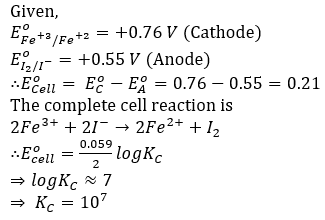Question 51. If an aqueous solution of NaF is electrolyzed between inert electrodes, the product obtained at the anode is:

1. a. Na
2. b. O2
3. c. F2
4. d. H2

Solution:

At the anode, oxidation takes place. Here water will be oxidized. The reaction at the anode will be:

2H2 O → 4H+ + O2 + 4e-

Question 52. In which of the following cases, a chemical reaction is possible:

1. a. Conc. HNO3 is stored in a platinum vessel
2. b. Gold ornaments are washed with dil. HCl
3. c. ZnSO4 is placed in a copper vessel
4. d. AgNO3 solution is stirred with a copper spoon

Solution:

Platinum and gold lie at the bottom of the reactivity series. Thus it does not react with HNO3 and HCl. Again, Zn is more reactive than copper. Thus, copper will be displaced by Zn, if ZnSO4 is placed in a copper vessel. But Ag+ is less reactive than copper. Thus, copper will displace silver. The corresponding reaction is: 2AgNO3 + Cu→Cu(NO3)2 + 2Ag

Question 53. The time required for 60% completion of a first-order reaction is 50 min. The time required for 93.6% completion of the same reaction will be

1. a. 50 min
2. b. 150 min
3. c. 100 min
4. d. 83.8 min

Solution: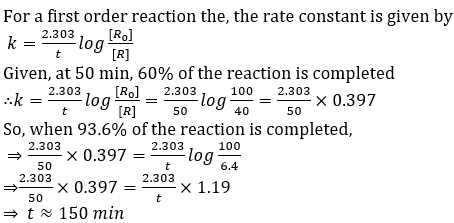Question 54. For an elementary reaction, 2A+3B→4C+D, the rate of appearance of C at a time `t’ is 2.8×10-3 molL-1 S-1. Rate of disappearance of B at `t’ will be:

1. a. 2(2.8×10-3) molL-1 S-1
2. b. 1/4(2.8×10-3) molL-1 S-1
3. c. 4/3(2.8×10-3) mol L-1 S-1
4. d. 3/4(2.8×10-3) mol L-1 S-1

Solution:

The given reaction is,

2A+3B -> 4C+D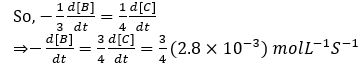Question 55. The rate constant of a reaction is given by k = PZe(-Ea/RT) under standard notation. In order to speed up the reaction, which of the following factors has to be decreased?

1. a. Ea
2. b. T
3. c. Z
4. d. Both Z and T

Solution:

The expression of rate constant given in the question ( k=PZ e(-Ea/RT)) is according to Arrhenius theory. To speed up the reaction, we will have to decrease the value of Ea i.e. activation energy and will have to increase the value of temperature (T) and the number of collisions (Z).

Question 56. A sol of AgI is prepared by mixing equal volumes of 0.1 M AgNO3 and 0.2 M KI, which of the following statement is correct?

1. a. Sol obtained is a positive sol with K+ adsorbed on AgI
2. b. Sol obtained is a negative sol with I- adsorbed on AgI
3. c. Sol obtained is a negative sol with NO3- adsorbed on AgI
4. d. Sol obtained is a positive sol with Ag+ adsorbed on AgI

Solution:

Given reaction is,

AgNO3+KI→ AgI(Sol)+KNO3

Here, the amount of AgNO3 present is 0.1 M.

Amount of KI present is 0.2 M.

Since KI is excess here, thus, sol obtained is a negative sol with I- adsorbed on AgI.

Question 57. During adsorption of a gas on a solid:

1. a. ∆G < 0, ∆H < 0, ∆S > 0
2. b. ∆G < 0, ∆H > 0, ∆S > 0
3. c. ∆G < 0, ∆H < 0, ∆S < 0
4. d. ∆G > 0, ∆H > 0, ∆S > 0

Solution:

When a gas is adsorbed on a solid surface, its movement is restricted leading to a decrease in the entropy of the gas i.e. ∆S is negative. Now, we know that, for a process to be spontaneous, ∆G should be negative.

We know, ∆G = ∆H-T∆S

Since ∆S is negative, ∆H has to be negative so as to make ∆G negative. Hence adsorption is always an exothermic process. Hence the correct option is (C).

Question 58. Copper is extracted from copper pyrites by:

1. a. Electrometallurgy
2. b. Auto reduction
3. c. Thermal decomposition
4. d. Reduction by coke

Solution:

The formula of copper pyrites is CuFeS2. In auto reduction, the sulphide ores of the metals which are less electropositive like Hg, Pb and Cu are heated in the air so as to convert a part of it to its oxides. These oxides then react with the remaining sulphide ore in the absence of air, to give the metal and sulphur dioxide. The corresponding reaction is:

Cu2 O+(1/2)Cu2 S→3Cu+(1/2)SO2

Question 59. Function of potassium ethylxanthate in froth floatation process is to make ore:

1. a. Hydrophilic
2. b. Heavier
3. c. Lighter
4. d. hydrophobic

Solution:

The function of potassium ethylxanthate in froth floatation process is to make the ore water repellant, i.e. hydrophobic.

Question 60. Sulphide ore on roasting gives a gas X. X reacts with Cl2 in the presence of activated charcoal to give Y. Y is:

1. a. SCl6
2. b. SOCl2
3. c. SO2Cl2
4. d. S2Cl2

Solution:

The corresponding reactions are;

2Cu2S+3O2→ 2 Cu2O + 2SO2 (s) (Reaction involved in roasting)

SO2+Cl2+ activatedcharcoal→SO2Cl2

So here X is SO2 (sulphur dioxide)

And Y is SO2Cl2 (Sulfuryl chloride)

### KCET 2020 Chemistry Question Paper with Solutions